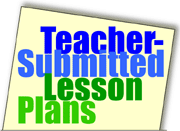Home >> A Tsl >> Archives >> 03 1 >> Equivalent Fraction Bingo

## Search formEquivalent Fraction Bingo

Subjects

• Applied Math
• Arithmetic
• Process Skills

• 3-5
• 6-8

Brief Description

This fun activity enhances students' understanding of equivalent fractions.

Objectives

Students
• recognize the value of equivalent fractions.
• use mathematical operations to determine equivalent fractions.
• use mathematical reasoning to discriminate fractions.

Keywords

fraction, math, Bingo, equivalent, numerator, denominator, whole

• paper (for fraction BINGO cards)
• fraction flash cards (see list of cards needed in lesson below)

The Lesson

Before introducing this game, review with students the concept of equivalent fractions. Have students list some fractions that are equivalent to 4/8 (for example, 1/2, 5/10, 3/6...).

Provide each student with a BINGO grid that is 3 squares by 3 squares (a total of nine squares) and have students write the following fractions in any order, one fraction per square.

• 6/36
• 2/10
• 4/16
• 3/9
• 10/25
• 2/4
• 8/12
• 6/8
• 9/9

The student's BINGO card might look like the one below:

 2/4 6/8 2/10 8/12 4/16 10/25 3/9 9/9 6/36

The teacher should make a deck of flash cards for her/himself. Each of the following fractions should appear on one of the flash cards:

• 1/2
• 3/4
• 1/5
• 2/3
• 1/4
• 2/5
• 1/3
• 1 (a whole)
• 1/6

The first student to fill up the 3-column by 3-row fraction card wins the game.

Extend the activity. When students have mastered the format of the game, increase the number of columns and rows on the fraction card and also increase the challenge by using higher fraction equivalence. You can adapt Education World's Math Bingo Card for this activity.

Assessment

Students will make the correct correlations between equivalent fractions. They might write in their math journals an explanation of the strategies they used to find equivalent fractions.

Submitted By

Raymundo V. De Jesus, Harriet Tubman Learning Center (P.S.154M) in New York City

Education WorldÂ®